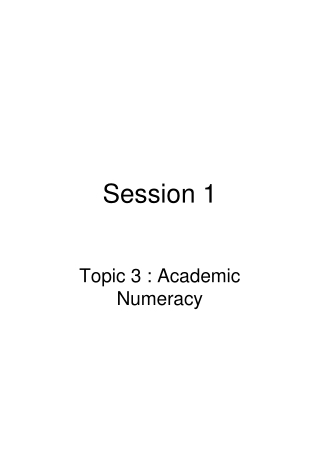DownloadDownload PresentationSession 1

# Session 1

Download Presentation## Session 1

- - - - - - - - - - - - - - - - - - - - - - - - - - - E N D - - - - - - - - - - - - - - - - - - - - - - - - - - -
##### Presentation Transcript

1. Session 1 Topic 3 : Academic Numeracy

2. Introduction • Tony D Susanto (Tony) PhD Student – Computer email: susa0004@flinders.edu.au Web: tonyteaching.wordpress.com --------------------------------------------------------- 1. Now, Get information about person next to you (name, address, height, weight,…)  You should describe about that person later (as clear as possible) --------------------------------------------------------- QUANTITATIVE Information e.g. 30 years old, 160 cm, has 2 sons INFORMATION QUALITATIVE Information e.g. tall, beautiful, brown eyes, curly hair

3. 2. Mention one of your daily activities that requires mathematical skill ! • Understanding the place values of number Hundreds of Thousands Thousands  Tens of Thousands   Hundreds  Tens  Units  Millions 8,901,634 (put coma in groups of three digits) • Meaning of + and - e.g. +5 or often written as 5, - 3 + and - are shown position from zero value Positive value is quantities more than zero Zero value is Reference point ~ Sea level ~ Starting point ~ You don’t have any money & don’t have any debt 2 0 -5 -4 -3 -2 -1 1 2 3 4 5 1 0 -1 -2 Negative value is quantities less than zero

4. Basic Operations: Addition (+), Subtraction (-), Multiplication (x), Division (/), Power, and Roots (√ ) 1. Addition (+) is combining two numbers. A + B = B + A A + (-B) = A - B e.g.: 3 + 2 = 2 + 3 3 + (-2) = 3 - 2 2. Subtraction (-) is taking one number from another. A - B ≠ B - A A - B = A + (-B) e.g.: 3 - 2 ≠ 2 - 3 3 - 2 = 3 + (-2)

5. Brackets ( ) { } [ ] “all inside the brackets are one unit so do it first!” e.g.: 10 + (2 – 1) = 10 + 1 = 11 10 x (2 – 1) = 10 x 1 = 10 Nested Brackets (10 x (2 – 1)) x 2 = (10 x 1) x 2 = 10 x 2 = 20 3. Multiplication x *  3 x 2 multiplying 3 with 2 means “adding 2 units of 3” = = 6 + A x B = B x A A x 1 = A A x 0 = 0 A x (B x C) = ( A x B ) x C = A x B x C e.g.: 3 x 2 = 2 x 3 1000 x 1 = 1000 19878 x 0 = 0 3 x (2 x 4) = (3 x 2) x 4 = 3 x 2 x 4 = 24

6. 4. Division  / ‒ “To share some quantity and then calculate the size of each share” Is the inverse of multiplication 3  2 = 3 / 2 = 3 2 Dividing by zero is not permissible (not available) 3  0 = no result Rules for Multiplication and Division when working with positive or negative numbers Same signs (all number are positive or all numbers are negative) = Positive answers Different signs (a number is positive and the other is negative) = Negative answers 3 x (-2) = -6 6 / 2 = 3 -3 x (-2) = 6 (-6) / 2 = -3 3 x 2 = 6 6 / (-2) = 3

7. 5. Power “multiplying a number by itself one or more times (based on the exponent)” 32 = 3 x 3 = 9 • = 2 x 2 x 2 x 2 = 16 Any number raised to the power of zero is 1 n0=1 e.g. 1987670=1 10=1 Negative Exponents a-n= =an 1 an 1 a-n n 6. Roots  Inverse of Powers 16 = ? ?2 = 16 “What number that if multiplied by itself twice will get 16” 27 = ? ?3 = 27 “What number that if multiplied by itself three times will get 27” 3

8. Order of Operation • BEDMAS: • Brackets • Exponents • Division • Multiplication • Addition • Subtraction